# Expanding Brackets

Here we break down everything you need to know about expanding brackets. You’ll learn how to expand single brackets and double brackets in order to leave a simplified algebraic expression.

At the end you’ll find expanding brackets worksheets based on Edexcel, AQA and OCR exam questions, along with further guidance on where to go next if you’re still stuck.

## What does expanding brackets mean?

To expanding brackets means multiplying each term in the brackets by the expression outside the brackets.

Expanding brackets is the reverse process of factorisation and is sometimes referred to as multiplying out. In effect by expanding brackets you are removing the brackets.

To expand brackets we multiply everything outside of the bracket, by everything inside the bracket. For example if we expand

$(2x + 1)(x − 3)$

We will get

$(2x + 1)(x − 3) = 2x^{2} − 5x − 3$

### What does expanding brackets mean?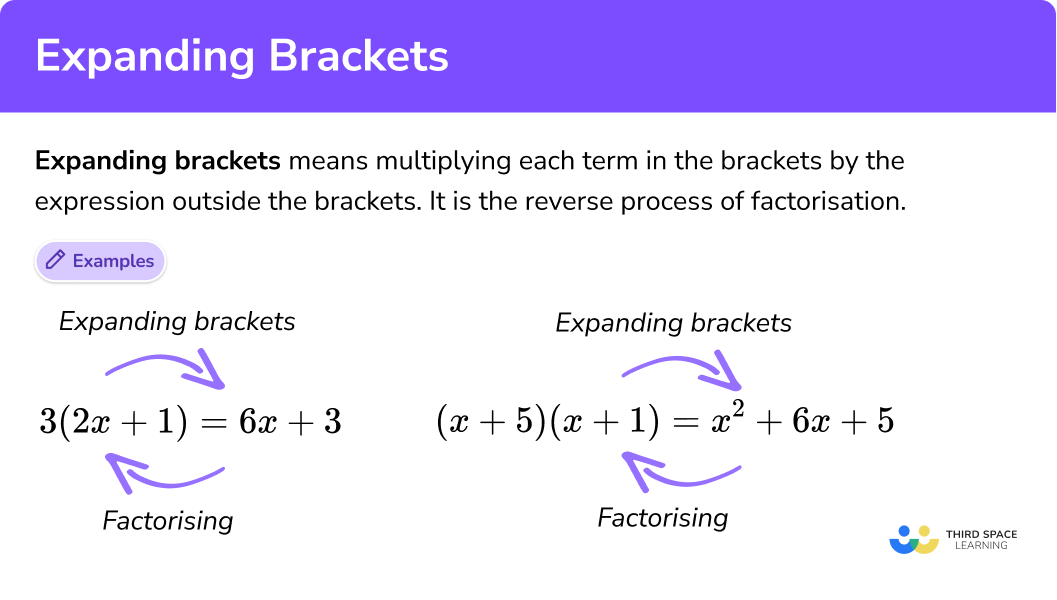This lesson is part of our series of lessons to support revision on algebraic expressions. Related step by step guides include:

## Multiplying out brackets

‘Multiplying out brackets’ or ’multiply out’ is another term for expanding brackets. It means exactly the same thing. “Expand the brackets” is the same as “multiply out the brackets”, it just gives the additional clue that when we expand brackets, we are multiplying everything outside the brackets by everything inside the brackets.

### Use of brackets

One use of brackets in maths in maths is to group items together, another is to give information about the order of operations.

E.g

Here is a rectangle.

Its perimeter is:

(x+8)+(x-3)+(x+8)+(x-3)

Here the brackets are used to group the terms so that the expressions for the sides are clear.

The perimeter can also be written as:

2(x+8)+2(x-3)

Here brackets are needed to preserve that the whole expressions for the sides are doubled to find the perimeter of a rectangle.

## How to expand brackets

In order to expand brackets you need to multiply the terms outside the brackets (or parentheses) by the terms inside the brackets. There are three main ways to do this, each of which is explained below.

• Expanding single brackets
• Expanding double brackets
• Expanding triple brackets

### How to expand brackets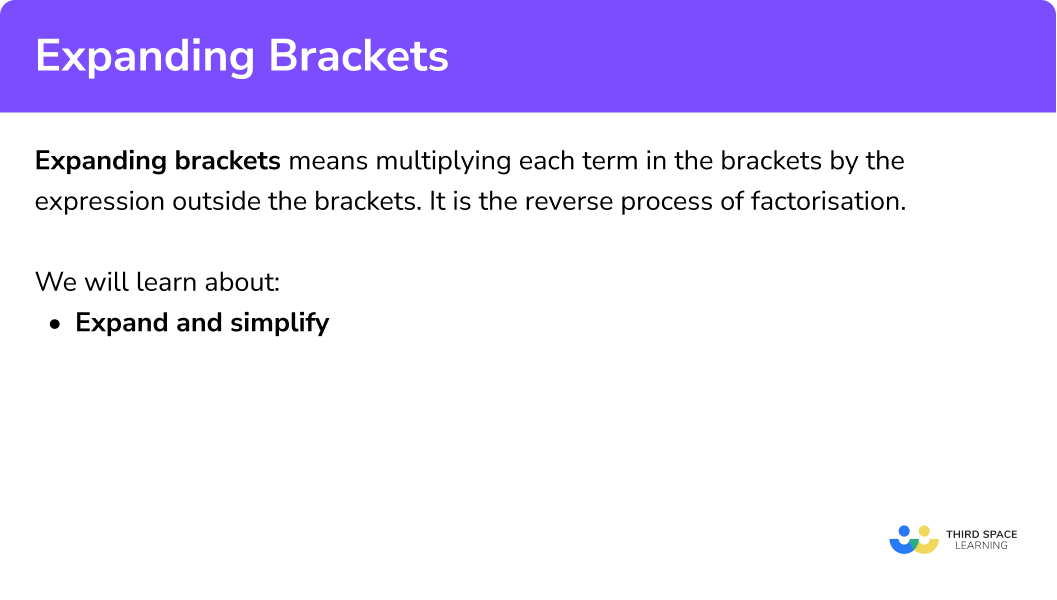1. Expanding single brackets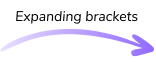3(2x + 1) = 6x + 3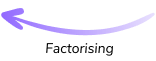Expressions with two terms like 6x + 3 are known as a binomials.

2. Expanding double brackets(x + 5)(x – 1) = x2+ 4x – 5Expressions with three terms like x2 + 4x − 5 are known as trinomials.

Terms that are raised to the power of 2 like x2 are known as quadratic terms.

3. Expanding triple brackets(x + 1)(x + 2)(x + 3) = x3 + 6x + 11x + 6A polynomial expression consists of two or more algebraic terms.

### Expanding brackets with surds

There is a fourth, more complex situation in which you may need to use your expanding brackets knowledge but this is usually only seen on the higher level GCSE maths papers so is not part of this lesson.

In summary, in order to expand brackets involving surds we multiply every term outside the brackets by every term inside the brackets and follow the rules of surds.

For example if we expand

$\sqrt{5}(\sqrt{3} – 2\sqrt{5})$

We will get

$\sqrt{5}(\sqrt{3} – 2\sqrt{5}) = \sqrt{15} – 10$

Step-by-step guide: Surds

## 1) Expanding single brackets

To expand a single bracket we multiply the term outside of the bracket by everything inside of the bracket.

## How to expand single brackets

In order to expand single brackets:

1. Multiply the term outside of the bracket by the first term inside the bracket.
2. Multiply the term outside the bracket by the second term inside the bracket.

### How to expand single brackets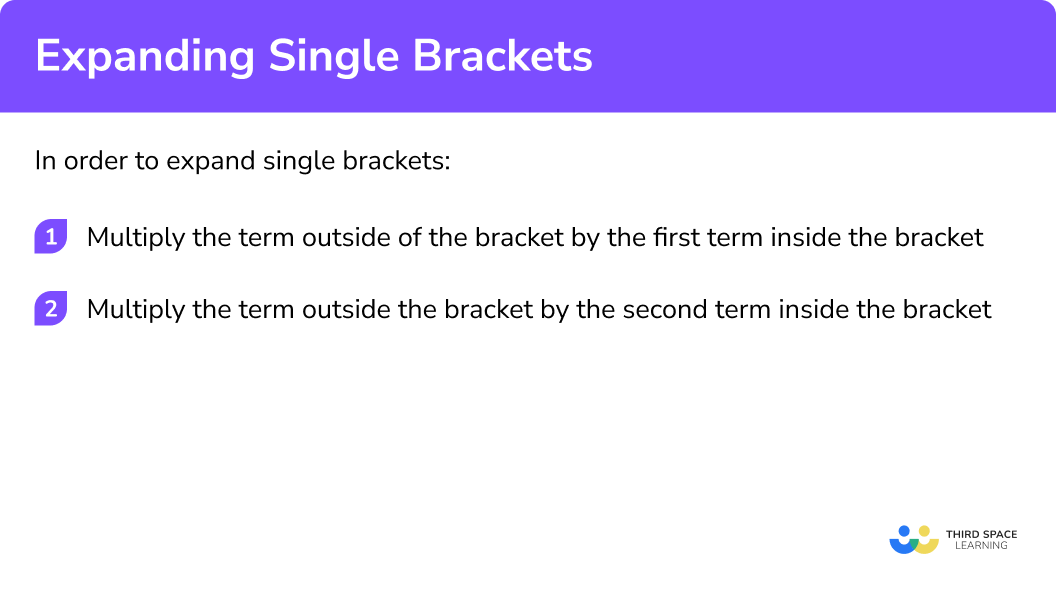## Expanding brackets examples (single brackets)

### Example 1: two terms in the bracket

Expand:

2(x + 3)

1. Multiply the term outside of the bracket (2) by the first term inside the bracket (x).

2 ✕ x = 2x

2Multiply the value outside the bracket (2) by the second term inside the bracket (3).

2 ✕ 3 = 6

The answer is positive so we need to write + 6.

2(x + 3) = 2x + 6

### Example 2: two terms in the bracket and a negative term outside

Expand:

− 3(y − 4)

− 3 ✕ y = − 3y

+ = so the answer is negative.

− 3 x − 4 = + 12

= + so the answer is positive. We need to write + 12.

− 3(y − 4) = − 3y + 12

### Example 3: two terms in the bracket and variables with coefficients greater than 1

Expand:

3x(4x − 2y)

3x ✕ 4x = 12x2

3x ✕ − 2y = − 6xy

+ = so the answer is negative. We need to write − 6xy.

3x(4x − 2y) = 12x2 − 6xy

### Example 4: three terms in the bracket and variables with coefficients greater than 1

Expand:

2x(3 − 5y + 6x2)

2x ✕ 3 = 6x

2x ✕ − 5y = − 10xy

+ = so the answer is negative. We need to write 10xy.

2x ✕ 6x2 = + 12x3

The answer is positive so we need to write + 12x3.

$2x( 3 – 5y + 6x^2) =$
$6x – 10xy + 12x^3$

## Practice expanding brackets questions (single brackets)

1. Expand: 6(y-2)

6y-2y-126y-12-12yWith a single bracket expansion, we must be sure to multiply each term inside the bracket by the number in front of the bracket.

2. Expand: -2(x+6)

2x+12-2x+12-2x-6-2x-12With a single bracket expansion, we must be sure to multiply each term inside the bracket by the number in front of the bracket. Care must be taken when multiplication involves negative numbers.

3. Expand: y(2y-3)

2y-32y^{2}-35y^{2}2y^{2}-3yWith a single bracket expansion, we must be sure to multiply each term inside the bracket by the number in front of the bracket. Make sure to include the correct index numbers.

4. Expand: -5x(2x+4)

-10x^{2}+20x10x^{2}-20x-10x^{2}-20x10x^{2}+20xWith a single bracket expansion, we must be sure to multiply each term inside the bracket by the number in front of the bracket. Make sure to include the correct index numbers. Care must be taken when multiplication involves negative numbers.

5. Expand: 4(8-3x+2y)

32-12x-8y32+12x+8y32-12x+8y32-3x+2yWith a single bracket expansion, we must be sure to multiply each term inside the bracket by the number in front of the bracket.

6. Expand: 3y(2+7y-4x)

6y+21y^{2}+12xy6y+21y-12xy6y+21y^{2}-12xy6+21y^{2}-12xyWith a single bracket expansion, we must be sure to multiply each term inside the bracket by the number in front of the bracket. Make sure to include the correct index numbers.

## 2) Expanding double brackets

To expand double brackets we multiply every term in the first bracket, by every term in the second bracket.

## How to expand double brackets

In order to expand double brackets follow these steps:

1. Draw a grid and insert the terms of the first and second brackets.
2. Fill in the grid by multiplying each of the terms together.
3. Write out each of the terms and simplify the expression by collecting like terms.

### How to expand double brackets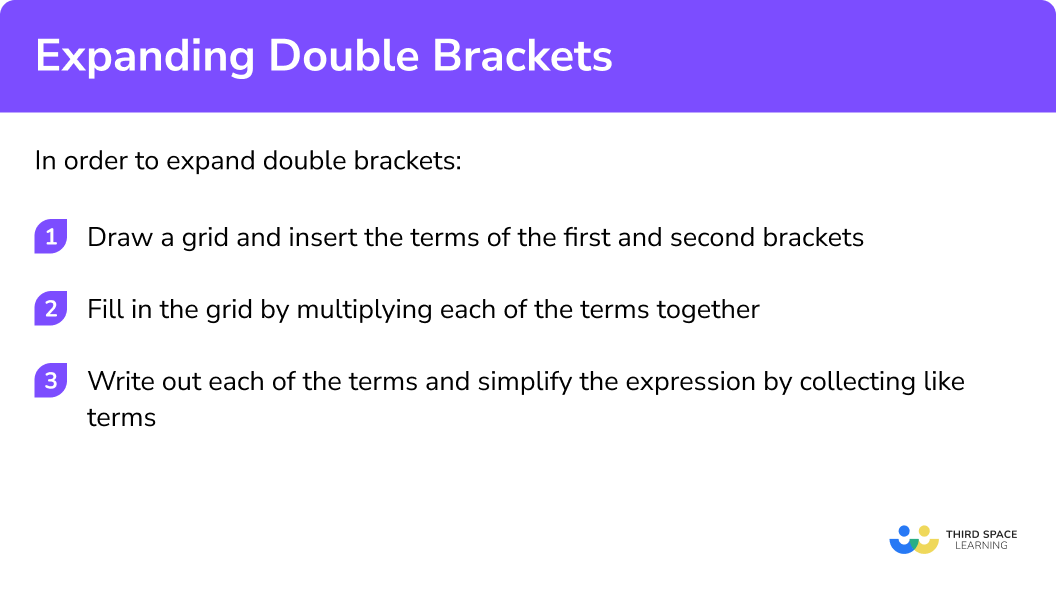## Expanding brackets examples (double brackets)

### Example 1: variables have a coefficient of 1 and there is + in both brackets

Expand and simplify:

(x+2)(x+3)

1. Draw a grid and insert the terms of the first and second brackets.

2Fill in the grid by multiplying each of the terms together.

x ✕ x = x2
x ✕ 3 = 3x
x ✕ 2 = 2x
2 ✕ 3 = 6

3Write out each of the terms and simplify the expression by collecting like terms.

x2 + 3x + 2x + 6
= x2 + 5x + 6

### Example 2: variables have a coefficient of 1 and there is + in one bracket and – in the other

Expand and simplify:

(x + 5)(x − 1)

x ✕ x = x2
x ✕ − 1 = − x

+ = so the answer is negative.

x ✕ 5 = 5x
5 ✕ − 1 = − 5

+ = so the answer is negative.

x2 − x + 5x − 5
x2 + 4x − 5

### Example 3: variables have a coefficient greater than 1 and there is + in one bracket and – in the other

Expand and simplify:

(2x − 3)(x + 4)

2x ✕ x = 2x2
2x ✕ 4 = 8x
x ✕ − 3 = − 3x

+ = so the answer is negative.

4 ✕ − 3 = − 12

+ = so the answer is negative.

2x2 + 8x − 3x − 12
2x2 + 5x − 12

### Example 4: squared brackets

Expand and simplify:

(3x − 4)2

(3x − 4)2 = (3x − 4)(3x − 4)

Remember: when we square something (raise it to the power of 2) we multiply it by itself.

3x ✕ 3x = 9x2
3x ✕ − 4 = − 12x
3x ✕ − 4 = − 12x

+ = so the answer is negative.

− 4 ✕ − 4 = + 16

= + so the answer is positive.

9x2 − 12x − 12x + 16
9x2 − 24x + 16

## Practice expanding brackets questions (double brackets)

1. Expand and simplify: (x+5)(x+6)

2x+11x^{2}+11x+30x^{2}+5x+30x^{2}+6x+30(x+5)(x+6)

Expanding the brackets gives

x^{2}+6x+5x+30

and then we can collect like terms

x^{2}+11x+30

2.Expand and simplify: (x-4)(x+2)

x^{2}-2x+8x^{2}-6x-8x^{2}-2x-8x^{2}+2x-8(x-4)(x+2)

Expanding the brackets gives

x^{2}+2x-4x-8

and then we can collect like terms

x^{2}-2x-8

3.Expand and simplify: (2x+3)(x+4)

2x^{2}+8x+12x^{2}+8x+12x^{2}+11x+122x^{2}+11x+12(2x+3)(x+4)

Expanding the brackets gives

2x^{2}+8x+3x+12

and then we can collect like terms

2x^{2}+11x+12

4. Expand and simplify: (3x-2)(x+1)

3x^{2}+x-23x^{2}-x-23x^{2}+x+2x^{2}+x-2(3x-2)(x+1)

Expanding the brackets gives

3x^{2}+3x-2x-2

and then we can collect like terms

3x^{2}+x-2

5. Expand and simplify: (x-4)^{2}

x^{2}-4x+16x^{2}-8x+16x^{2}-8x-16x^{2}+8x+16(x-4)^{2}

means the same as

(x-4)(x-4)

so we can expand as usual

x^{2}-4x-4x+16

and then collect like terms

x^{2}-8x+16

6.Expand and simplify: (2x+5)^{2}

2x^{2}+20x+252x^{2}+10x+254x^{2}+20x+252x^{2}+10x+5(2x+5)^{2}

means the same as

(2x+5)(2x+5)

so we can expand as usual

4x^{2}+10x+10x+25

and then collect like terms

4x^{2}+20x+25

## 3) Expanding triple brackets

To expand triple brackets we first multiply the first two brackets together. We then multiply every term in this new expression by every term in the third bracket.

## How to expand triple brackets

In order to expand triple brackets:

1. Draw a grid, insert the terms of the first and second brackets, then fill it in by multiplying each of the terms together.
2. Write out each of the terms and simplify the expression by collecting like terms.
3. Draw a grid, insert the terms from this new expression and the third bracket, then fill it in by multiplying each of the terms together.
4. Write out each of the terms and simplify the expression by collecting like terms.

### How to expand triple brackets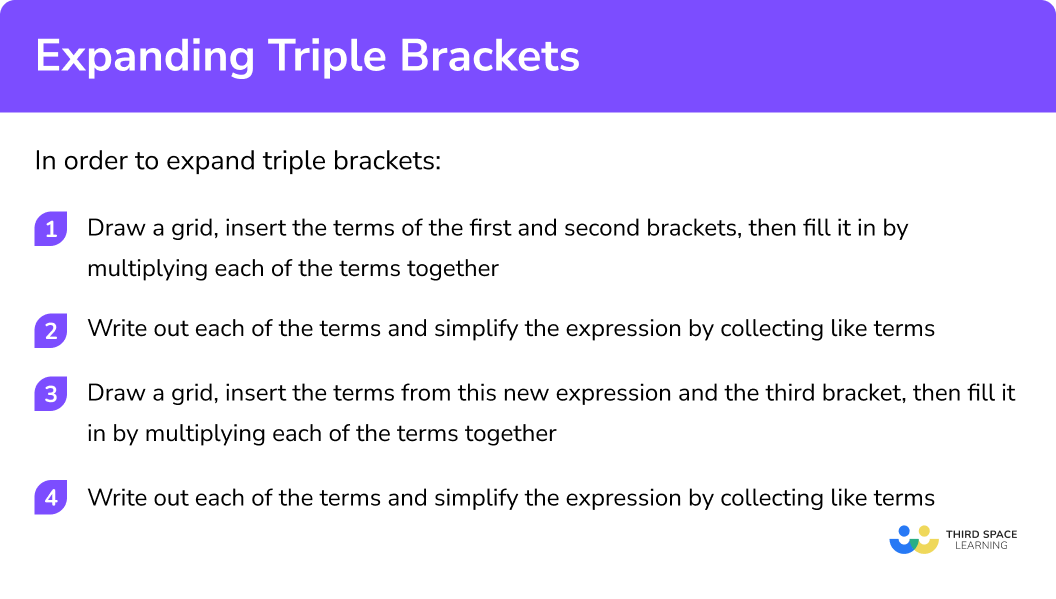## Expanding brackets examples (triple brackets)

### Example 1: multiply three brackets

Expand and simplify:

(x + 1)(x + 2)(x + 3)

1. Draw a grid, insert the terms of the first and second brackets, then fill it in by multiplying each of the terms together.

x ✕ x = x2
x ✕ 2 = 2x
x ✕ 1 = x
1 ✕ 2 = 2

2Write out each of the terms and simplify the expression by collecting like terms.

x2 + 2x + x + 2
x2 + 3x + 2

3Draw a grid, insert the terms from this new expression and the third bracket, then fill it in by multiplying each of the terms together.

x ✕ x2 = x3
x ✕ 3x = 3x2
x ✕ 6 = 6x
3 ✕ x2 = 3x2
3 ✕ 3x = 9x
3 ✕ 6 = 18

4Write out each of the terms and simplify the expression by collecting like terms.

x3 + 3x2 + 3x2 + 9x + 2x + 6
x3 + 6x2 + 11x + 6

### Example 2: squared brackets multiplied by a third bracket

Expand and simplify:

(x + 3)2(x − 1)

(x + 3)2 = (x + 3)(x + 3)

x ✕ x = x2
x ✕ 3 = 3x
x ✕ 3 = 3x
3 ✕ 3 = 9

x2 + 3x + 3x + 9
x2 + 6x + 9

x ✕ x2 = x3
x ✕ 6x = 6x2
x ✕ 9 = 9x
− 1 ✕ x2 = − x2
− 1 ✕ 6x = − 6x
− 1 ✕ 9= − 9

x3 + 6x2 − x2 + 9x − 6x − 9
x3 + 5x2 + 3x − 9

## Practice expanding brackets questions (triple brackets)

1. Expand and simplify: (x+2)(x+3)(x+4)

x^{3}+9x^{2}+9x+24x^{3}+9x^{2}+24x+26x^{3}+9x^{2}+26x+24x^{3}+7x^{2}+24x+24(x+2)(x+3)(x+4)

Expanding the first two brackets gives

(x^{2}+3x+2x+6)(x+4)

or

(x^{2}+5x+6)(x+4)

and then expanding again

x^{3}+4x^{2}+5x^{2}+20x+6x+24

Collect like terms

x^{3}+9x^{2}+26x+24

2. Expand and simplify: (x+3)(x-2)^2

x^{3}-x^{2}-8x+12x^{3}+x^{2}-8x+12x^{3}-x^{2}+8x+12x^{3}-x^{2}-8x+6(x+3)(x-2)^{2}

can be written as

(x+3)(x-2)(x-2)

Expanding the two identical brackets first gives

(x+3)(x^{2}-2x-2x+4) (x+3)(x^{2}-4x+4)

and expanding again

x^{3}-4x^{2}+4x+3x^{2}-12x+12

then collect like terms

x^{3}-x^{2}-8x+12

3.Expand and simplify: (2x+1)^3

8x^{3}+8x^{2}+6x+18x^{3}+12x^{2}+12x+18x^{3}+12x^{2}+6x+12x^{3}+12x^{2}+6x+1(2x+1)^{3}

can be written as

(2x+1)(2x+1)(2x+1)

Expanding two of the brackets gives

(2x+1)(4x^{2}+2x+2x+1)

(2x+1)(4x^{2}+4x+1)

and expanding again

8x^{3}+8x^{2}+2x+4x^{2}+4x+1

then collect like terms

8x^{3}+12x^{2}+6x+1

## Common misconceptions

• We must multiply the value outside the brackets by every term inside the brackets.
2(6x2 − 3x) = 12x2 − 3x  ✖

Here we have multiplied the value outside of the brackets by the first term inside of the bracket, but not the second term.

The correct answer is 2(6x2 − 3x) = 12x2 − 6x

We need to multiply all the terms inside the bracket.

• For two numbers to multiply to give a + their signs must be the same.

+ ✕ + = +
− ✕ − = +

e.g. 2 ✕ 3 = 6
e.g. − 2 ✕ − 3 = 6

4 ✕ 5 = 20
− 4 ✕ − 5 = 20

• For two numbers to multiply to give a their signs must be different.

+ ✕ − = −
− ✕ + = −

e.g. 2 ✕ − 3 = − 6
e.g. − 2 ✕ 3 = − 6

4 ✕ − 5 = − 20
− 4 ✕ 5 = − 20

− 4(3y − 5) = − 12y − 20 ✖

Here we have not used = +

− 4 ✕ − 5 = + 20

The correct answer is − 4(3y − 5) = − 12y + 20

• When we square something, we multiply it by itself.
32 = 3 ✕ 3
x2 = x ✕ x
(5y)2 = 5y ✕ 5y

• When we square a bracket, we multiply it by the entire bracket.
(x + 3)2 = (x + 3)(x + 3) ✔
NOT x2 + 9 ✖

## Expanding brackets GCSE questions

1. Expand: 3(x – 2)

3x – 6

(1 mark)

2. Expand: 4x(2x – 7)

8x2 – 28x

(1 mark)

3. Expand and simplify: 5(x – 3) – 3(x + 5)

2x – 30

(2 marks)

## Learning checklist

You have now learned how to:

• Multiply a single term over a bracket
• Expand products of 2 or more binomials

## Still stuck?

Prepare your KS4 students for maths GCSEs success with Third Space Learning. Weekly online one to one GCSE maths revision lessons delivered by expert maths tutors.

Find out more about our GCSE maths tuition programme.